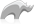##### [ bytes ]

Method

[Bytes] creates a byte stream that can be used to work with binary data or to convert strings from one character set to another. The [Bytes] method can be used to instantiate a new bytes object, or it can be used to cast string data types as bytes types. Only string data types may be cast as bytes data types.

In the first form the method accepts two optional parameters. The first parameter is a string to be imported into the byte stream. The second parameter defines what character set the string is transformed into before it is imported (e.g. iso-8859-1). If no character set is specified then the string is imported as UTF-8.

In the second form the method accepts two optional parameters. The first specifies the size of the memory buffer in bytes that should be allocated to hold the byte stream. The second specifies the number of bytes that should be added if the initial memory buffer is too small to hold the entire byte stream.

• Syntax
• Methods
• Traits
``````local(Bytes_Import = Bytes('String Value'))

local(Bytes_ISO_Import = Bytes('String Value', 'iso-8859-1'))

local(Bytes_Preallocate = Bytes(1024))

local(Bytes_Growth = Bytes(1024, 256))``````
###### Has methods:
• `bytes->oncompare(p0::bytes)`
• `bytes->oncompare(s::string)`
• `bytes->setsize(p0::integer)`
• `bytes->append(p0::bytes)`
• `bytes->append(rhs::string)`
• `bytes->private_find(p0::bytes, p1::integer, p2::integer, p3::integer, p4::integer)`
• `bytes->reserve(p0::integer)`
• `bytes->getrange(p0::integer, p1::integer)`
• `bytes->sub(p0::integer, p1::integer)`
• `bytes->sub(pos::integer)`
• `bytes->private_setrange(p0::bytes, p1::integer, p2::integer, p3::integer)`
• `bytes->importas(p0::string, p1::string)`
• `bytes->import8bits(p0::integer)`
• `bytes->import32bits(p0::integer)`
• `bytes->import64bits(p0::integer)`
• `bytes->import16bits(p0::integer)`
• `bytes->importbytes(p0::bytes)`
• `bytes->importpointer(p0::integer, p1::integer)`
• `bytes->export8bits()`
• `bytes->export16bits()`
• `bytes->export32bits()`
• `bytes->export64bits()`
• `bytes->exportbytes(p0::integer)`
• `bytes->exportsigned8bits()`
• `bytes->exportsigned16bits()`
• `bytes->exportsigned32bits()`
• `bytes->exportsigned64bits()`
• `bytes->trim()`
• `bytes->marker()`
• `bytes->marker=(p0::integer)`
• `bytes->remove(p0::integer, p1::integer)`
• `bytes->remove()`
• `bytes->swapbytes()`
• `bytes->size()`
• `bytes->length()`
• `bytes->encodeurl(p0::boolean)`
• `bytes->encodeurl()`
• `bytes->decodeurl()`
• `bytes->encodebase64()`
• `bytes->decodebase64()`
• `bytes->encodeqp()`
• `bytes->decodeqp()`
• `bytes->encodemd5()`
• `bytes->encodehex()`
• `bytes->decodehex()`
• `bytes->uncompress()`
• `bytes->compress()`
• `bytes->detectcharset()`
• `bytes->bestcharset(p0::string)`
• `bytes->crc()`
• `bytes->asString()`
• `bytes->oncreate(initial::integer)`
• `bytes->oncreate(copy::bytes)`
• `bytes->oncreate(import::string)`
• `bytes->oncreate(import::string, ENCODING::string)`
• `bytes->oncreate(import)`
• `bytes->importString(s::string, enc::string = ?)`
• `bytes->+(rhs::bytes)`
• `bytes->+(rhs::string)`
• `bytes->find(find::bytes, position::integer = ?, length::integer = ?, patPosition::integer = ?, patLength::integer = ?)`
• `bytes->find(find::string, position::integer = ?, length::integer = ?, patPosition::integer = ?, patLength::integer = ?)`
• `bytes->contains(find)`
• `bytes->get(position::integer)`
• `bytes->beginsWith(find::string)`
• `bytes->beginsWith(find::bytes)`
• `bytes->endsWith(find::string)`
• `bytes->endsWith(find::bytes)`
• `bytes->padLeading(tosize::integer, with::bytes)`
• `bytes->padLeading(tosize::integer)`
• `bytes->padLeading(tosize::integer, s::string)`
• `bytes->padTrailing(tosize::integer, with::bytes)`
• `bytes->padTrailing(tosize::integer)`
• `bytes->padTrailing(tosize::integer, s::string)`
• `bytes->setrange(what::bytes, where::integer = ?, whatStart::integer = ?, whatLen::integer = ?)`
• `bytes->split(find::string)`
• `bytes->split(find::bytes)`
• `bytes->removeleading(find::bytes)`
• `bytes->removetrailing(find::bytes)`
• `bytes->replace(find::bytes, replace::bytes)`
• `bytes->replace(find::string, replace::string)`
• `bytes->exportAs(ENCODING::string = ?)`
• `bytes->exportString(ENCODING::string)`
• `bytes->exportPointerBits()`
• `bytes->forEachByte()`
• `bytes->eachByte()::trait_forEach`
• `bytes->setposition(i::integer)`
• `bytes->position=(i::integer)`
• `bytes->position()`
###### Has traits:
• `any`
• `trait_asString`
• `trait_scalar`
• `trait_serializable`
##### Examples

No examples foundPlease note that periodically LassoSoft will go through the notes and may incorporate information from them into the documentation. Any submission here gives LassoSoft a non-exclusive license and will be made available in various formats to the Lasso community.